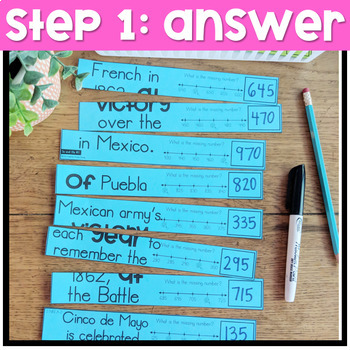# 2nd grade Math Review | End of Year Math Puzzles | Cut and Paste OptionSubject
Resource Type
Product Rating
4.0
6 Ratings
File Type

PDF (Acrobat) Document File

135 MB|125 pages
Share
Also included in:
1. These 2nd grade math centers and math interactive notebook cut and paste activities focus on the 2nd grade common core math standards and are perfect for guided math rotations or math workshop!________________________________Save money, buy the 2nd Grade bundle.3rd Grade bundle.This GROWING BUNDLE r
\$21.25
\$14.00
Save \$7.25
Product Description

Are you looking for the 3rd Grade End of the Year Math Puzzles?

©Unlock the Fact Math Puzzles are an original idea by The Teacher Wears Prada.

>>>>Click here to read a blog post about these Unlock the Fact Math Puzzles and see different examples of this resource, how it works, etc.

These math puzzles are perfect for end of the year math review and keeping your students engaged while their minds are thinking about all things summer!

How do these low-prep math puzzles work?

The MAY/End of the Year EDITION of these cross-curricular Unlock the Fact Math Puzzles reveal facts themed around holidays in May. Students will answer the 9 math problems, put the puzzle strips in order from LEAST to GREATEST. If they have answered the problems correctly and have placed the numbers in order correctly, an interesting fact will be revealed. They will have "unlocked a fact".

Content Integration + Self-Correcting Math Puzzles = Happy Teacher and Engaged Students

What themes/facts are featured this month?

Asian Pacific American Heritage Month, National Space Day (facts about Planets), National Astronaut Day (facts about Astronauts), National Tuna Day (facts about tuna), National Shrimp Day (facts about shrimp), National Bike Day (facts about history of bikes), National Train Day (facts about history of trains), Cinco de Mayo, Memorial Day, Kentucky Derby, National Egg Month, National Love a Tree Day (facts about trees)

This resource includes 50 different math puzzles {two different formats} that cover a variety of 2nd Grade Common Core Math Skills. A recording sheet for accountability and a fact booklet for students to record their facts {optional} are also included.

Two different formats included:

Option #1: {MATH CENTERS} Print on colored paper, laminate, students write and wipe the answers with a thin dry erase marker. These laminated puzzles can be placed in math centers.

OR

Option #2: {PRINT AND GO RESOURCE} Pieces are already mixed up/jumbled up for students to solve, cut and put together to glue into math notebook or on a piece of construction paper. Students can complete these independently for morning work, math warm-ups, independent math work during math rotations, homework etc.

What skills are included?

{The Common Core standard is printed in the upper left corner of each puzzle}

• Telling Time (Quarter Hour)
• Telling Time (Five Minutes)
• Counting Money (pennies, nickels, dimes, quarters)
• Money Word Problems
• Identifying Halves, Thirds, Fourths, Fifths, Sixths, Eights
• Identifying Fractions
• Fractions of a Set
• Estimating Weight (Metric and Customary)
• Word Problems (Addition and Subtraction)
• TWO STEP Word Problems
• Counting by 5s and 10s on a Number Line
• Counting by 2s
• Counting by 100s
• Before and After (3 Digit Numbers)
• Single Digit Addition and Subtraction Facts
• Mentally Add and Subtract 100 (Input Table)
• Even and Odd
• Plus 1, Minus 1, Plus 10, Minus 10 with 3 Digit Number
• 3 Digit Addition (No Regrouping)
• 3 Digit Subtraction (No Regrouping)
• 3 Digit Addition (With Regrouping)
• 3 Digit Subtraction (With Regrouping)
• Ordering Numbers (3 Digit Numbers)
• Comparing Numbers (3 Digit)
• Part Part Whole
• Missing Addend (one digit and two digit)
• Adding 10s {Example: 240 + 50 = }
• Subtracting 10s {Example: 270-30=}
• Bar Graph
• Line Plot
• Pictograph
• Fact Families
• Related Facts
• Place Value (3 Digit Numbers)
• Base Ten Blocks
• Regrouping Tens and Ones
• Identify the Value (3 Digit Numbers)
• Even and Odd Numbers (3 Digit Numbers)
• Word Form
• Expanded Form
• 2D and 3DShapes
• Multiplication (1s Facts)
• Multiplication (2s Facts)
• Arrays

___________________________________________________

Be the first to know about my new discounts, freebies and product launches:

If you're interested in more free resources, you can get access to my Free Resource Library here.

Connect with me:

Total Pages
125 pages